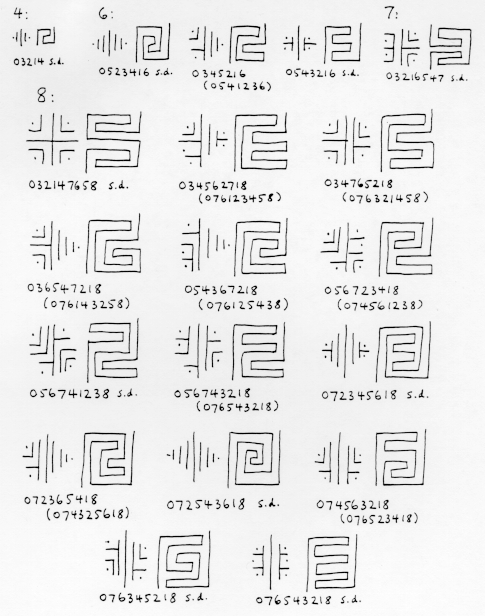Through Mazesto Mathematics

## A Combinatorial Problem: How many different n-level mazes are there?

Among the classical and medieval maze-types there are examples of different simple alternating transit mazes with the same number of levels. For instancethese three 12-level maze-paths all occur. This raises the question: How many different mazes are there with n levels?

Now it is possible to list all of the possible s.a.t. mazes of a given depth n, by looking at all the permutations of 0,...,n and discarding those which do not meet the three conditions which guarantee that a sequence corresponds to a maze. In fact, since odds and evens cannot mix in the permutation, and since 0 and n must stay fixed, it is enough to look at all pairs of permutations, one of the odd integers 1,...,n-1 (assuming n even), and one of the even integers 2,...,n-2. For example, to construct all possible 8-level mazes, one must consider pairs of permutations of 1,3,5,7 and of 2,4,6. There are 24 x 6 = 144 such pairs, so this can easily be done by hand. Here is what one finds for depths 1 through 8. In this list, the non-"interesting" mazes (those can be constructed from shallower mazes by adding trivial levels at the top and/or bottom) have been omitted. This figure shows the nuclei and rectangular mazepaths of these mazes; only one representative of each dual pair is drawn.The interesting mazes of 4,6,7 and 8 levels. Only one representative of each dual pair is drawn.
s.d. : self-dual.

Here are the data in tabular form:

```
Depth   Number of mazes    Interesting mazes

1              1                 none

2              1                 none

3              1                 none

4              2                03214

5              3                 none

6              8          0523416   0543216
0345216   0541236

7             14               03216547

8             42   032147658   034567218   034765218
036547218   054367218   056723418
056741238   056743218   072345618
072365418   072543618   074325618
074561238   074563218   076123458
076125438   076143258   076321458
076345218   076523418   076541238
076543218
```

For depth 9 one would have to consider 24 x 24 = 576 permutations, and the possibilities of error increase. The following numbers were calculated using computers.

```                    n           M(n)        I(n)
1             1          0
2             1          0
3             1          0
4             2          1
5             3          0
6             8          4
7            14          1
8            42         22
9            81         11
10           262        142
11           538         95
12          1828       1014
13          3926        808
14         13820       7796
15         30694       6980
16        110954      63386
17        252939      61725
18        933458     538534
19       2172830     558853
20       8152860    4740658
21      19304190    5171300
22      73424650   42969130

24     678390116

26    6405031050

28   61606881612

30  602188541928

32 5969806669034
```
Here M(n) and I(n) are the numbers of mazes and of interesting mazes of depth n. The values for 24, 26 and 28 were obtained by Jim Reeds of Bell Labs by a method which does not seem to apply to odd depth-numbers. The values for 30 and 32 are reported in Lando and Zvonkin's 1993 paper as due to V. R. Pratt.

The interesting 9-level mazes are shown this figure by their rectangular maze-paths; again, only one representative of each dual pair is drawn.The 11 interesting mazes of depth 9.

The next figure shows the maze paths of the 5 self-dual very-interesting mazes of depth 10; these are mazes which also have no internal sequences of trivial levels.The 5 very-interesting self-dual mazes of depth 10. The patterns labelled alpha_10 and gamma_10 occur in Roman mosaic mazes.
Added 11/18/2009 0321654987.10 should also be in this group. I am grateful to Walter D. Pullen for this information.

How are these numbers calculated?

Why did I get interested in this problem?

Where else do these numbers occur?

What else is known about the maze numbers?